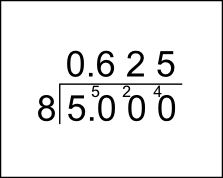The Singing Hedgehog Guide to:
Percentages - from fractions

There are three basic methods:

Firstly, turn into tenths, then into percentages:

3/5 = 6/10 = 60%

Second option, turn into hundredths,
then into percentages:

11/25 = 44/100 = 44%

Thirdly, we just have to divide:

5/8 = 5 ÷ 8    bus-stop time!0.625 = 62.5%

Now you need to practise: